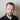# Sizing strategy boosting Sharpe ratio from 3.28 to 3.5

April 04, 2020 | strategy

I will be using one of the Bitcoin arbitrage strategies.

Sharpe ratio is a simple measure of risk adjusted returns, it’s not the best, but it will be sufficient here.

Original strategy generates Sharpe rato of 3.28 and max drawdown of 16%. That’s pretty good, but also makes 175% drawdown if reinvested:So, I decided to research this a little further.

Previously I sometimes was using one of the simplest switchers (shadows) on how to control drawdowns. It just takes average of the returns and trades only if returns are above it. But with arbitrage that won’t work, because you still need to wait until gap closes and such switchers can interfere in the wait.

It seems that Bitcoin returns are somewhat mean reverting…:Momentum Sharpe: 1.8759855761240092
Mean reversion Sharpe: 2.7845623552311247

…using this code:

from matplotlib import pyplot as plt

def get_sharpe(df, col, per):
return df[col].mean() / df[col].std() * sqrt(per)

def momentum_mean_reversion_test(df):
df['mom'] = where(df['returns'] > df['returns'].shift(), 1, 0)
df['mr'] = where(df['returns'] < df['returns'].shift(), 1, 0)
df['momret'] = df['mom'].shift() * df['returns']
df['mrret'] = df['mr'].shift() * df['returns']

momsharpe = get_sharpe(df, 'momret', 365)
mrsharpe = get_sharpe(df, 'mrret', 365)
print('Momentum Sharpe: {}'.format(momsharpe))
print('Mean reversion Sharpe: {}'.format(mrsharpe))

plt.plot(df['momret'].cumsum(), label='Momentum')
plt.plot(df['mrret'].cumsum(), label='Mean reversion')
plt.legend()
plt.show()

So, my simplest switcher may work if applied opposite way - trade only when returns are negative:

df['sma'] = df['returns'].rolling(window=10).mean()
# so, in order to modify the initial decision, we would look
# into previous day returns and nulify signals if returns
# are above the average:
df['sig'] = where(df['returns'] > df['sma'], 0, 1)
df['ret'] = df['sig'].shift() * df['returns']

Result:That makes 50% less over entire period and reduces max drawdown from 16% to 7%. So, we reduced income by 14% and reduced drawdown by 56%. Not bad at all:So, we now can run with reinvestment:Max drawdown of modified strategy is now 40% instead of 175%, approx. 77% less and returns over the entire period are approx. 56% lower.So, I hope this experiment shows a good deal on why it is so hard to reduce risk and not to reduce profit with it.

Note that reinvestment returns are misleading, because they largely depend on when you have started.